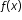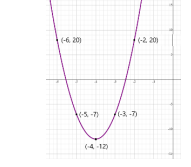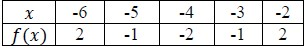# MA.912.AR.3.4Export Print
Write a quadratic function to represent the relationship between two quantities from a graph, a written description or a table of values within a mathematical or real-world context.

### Examples

Algebra I Example: Given the table of values below from a quadratic function, write an equation of that function.
 x -2 -1 0 1 22 -1 -2 -1 2

### Clarifications

Clarification 1: Within the Algebra 1 course, a graph, written description or table of values must include the vertex and two points that are equidistant from the vertex.

Clarification 2: Instruction includes the use of standard form, factored form and vertex form.

Clarification 3: Within the Algebra 2 course, one of the given points must be the vertex or an x-intercept.

General Information
Subject Area: Mathematics (B.E.S.T.)
Strand: Algebraic Reasoning
Status: State Board Approved

## Benchmark Instructional Guide

### Terms from the K-12 Glossary

• Coordinate Plane
• Domain
• Function Notation
• Range
• $x$-intercept
• $y$-intercept

### Vertical Alignment

Previous Benchmarks

Next Benchmarks

### Purpose and Instructional Strategies

In middle grades, students wrote linear two-variable linear equations. In Algebra I, students write quadratic functions from a graph, written description or table. In later courses, students will write quadratic two-variable inequalities.
• Instruction includes making connections to various forms of quadratic equations to show their equivalency. Students should understand when one form might be more useful than other depending on the context.
• Standard Form Can be described by the equation $y$ = $a$$x$ + $b$$x$ + $c$, where $a$, $b$ and $c$ are any rational number. This form can be useful when identifying the $y$-intercept.
• Factored Form Can be described by the equation $y$ = $a$($x$ − $r$1)($x$ − $r$2), where  $r$1 and  $r$2 are real numbers and the roots, or $x$-intercepts. This form can be useful when identifying the $x$-intercepts, or roots.
• Vertex Form Can be described by the equation $y$$a$($x$$h$)2 + $k$, where the point ($h$, $k$) is the vertex. This form can be useful when identifying the vertex.
• Instruction includes the use of $x$-$y$ notation and function notation.
• Instruction includes the connection to completing the square and literal equations to rewrite an equation from standard or factored form to vertex form.
• When determining the value of $a$ in a quadratic function, this can be done by two methods described below.
•  Students may notice a pattern from the points in the graph. When $a$ = 1, points 1 unit to the left or right of the vertex are 12 or 1 unit above or below the vertex. Points 2 units to the left or right of the vertex are (2)or 4 units above or below the vertex. Students may look at the table and notice this relationship exists, therefore, $a$ = 1.
• This process can be used for other values of $a$. When $a$ = 2, for example, points 1 unit to the left or right of the vertex are 2(12) or 2 units above or below the vertex. Points 2 units to the left or right of the vertex are 2(2)2 or 8 units above or below the vertex. Similarly, when $a$ = $\frac{\text{1}}{\text{2}}$, points 1 unit to the left or right of the vertex are $\frac{\text{1}}{\text{2}}$(12)  or $\frac{\text{1}}{\text{2}}$ a unit above or below the vertex. Points 2 units to the left or right of the vertex are $\frac{\text{1}}{\text{2}}$ (2)2 or 2 units 2 above or below the vertex.
• Students can solve for a by substituting the values of $x$ and $y$ from a point on the parabola.
• Ask students to consider $y$ = $a$($x$ + 4)2 − 2 and determine what information they would need to be able to solve for a. As students express the need to know values for $x$ and for $y$, ask them if they know any combinations of $x$ and $y$ that are solutions. Students could use a table of values or a graph, if given, to determine values that could be used for $x$ and $y$. Have students pick one and substitute and solve for $a$. Ask for students who chose different points to share their value for a to help them see that all points, when substituted, produce $a$ = 1.
• Instruction includes the use of graphing software or technology.
• For example, when determining the value of $a$, consider using graphing software to allow students to use sliders to quickly observe this. This provides opportunity for students to notice patterns regarding the value of $a$ and the concavity and stretch of the parabola (MTR.5.1).

### Common Misconceptions or Errors

• When writing functions in vertex form, students may confuse the sign of $h$
• For example, students may see a vertex of (−1, −2) and an $a$ value of 3 and write the function as $y$ = 3($x$ − 1)− 2 instead of $y$ = 3($x$ + 1)2 − 2. To address this, help students recognize that because $h$ is subtracted from $x$ in vertex form, it will change the sign of that coordinate. Show students a graph of both functions to confirm and make the connection to transformation of functions (MA.912.F.2.1).

### Strategies to Support Tiered Instruction

• Teacher models substituting roots into the factored form of a quadratic, $y$ = $a$($x$ $r$1)($x$$r$2). Instruction then includes reminding students of different methods that can be used to multiply binomials to convert the quadratic into standard form, $y$ = $a$$x$ + $b$$x$ + $c$
• Instruction includes solving for a by substituting the values of $x$ and $y$ from a point on the parabola. Students may need to review the order of operations to ensure they correctly isolate $a$. Provide students with a review of the order of operations.
• Parenthesis
• Exponents
• Multiplication or Division (Whatever comes first left to right)
• Addition or Subtraction (Whatever comes first left to right)
• Instruction includes recall of knowledge demonstrating how when $h$ is subtracted from $x$ in vertex form, it will change the sign of that coordinate. A graph of both functions can be used to confirm and make the connection to transformation of functions.
• Teacher provides a laminated cue card of the steps required to convert from factored form to standard form.

• Duane throws a tennis ball in the air. After 1 second, the height of the ball is 53 ft. After 2 seconds, the ball reaches a maximum height of 69 feet. After 3 seconds the height of the ball is 53 ft.
• Part A. Write a quadratic function to represent the height of the ball, $h$, at any point in time, $t$
• Part B. How long will the tennis ball stay in the air? Round your answer to the nearest tenth second.

• Part A. Create a quadratic function that contains the roots $\frac{\text{3}}{\text{5}}$ and 1.8.
• Part B. Compare your function with a partner. What do you notice?

### Instructional Items

Instructional Item 1
• Write the quadratic function that corresponds with the graph below.Instructional Item 2
• Given the table of values below from a quadratic function, write an equation of that function.*The strategies, tasks and items included in the B1G-M are examples and should not be considered comprehensive.

## Related Courses

This benchmark is part of these courses.
1200310: Algebra 1 (Specifically in versions: 2014 - 2015, 2015 - 2022, 2022 and beyond (current))
1200320: Algebra 1 Honors (Specifically in versions: 2014 - 2015, 2015 - 2022, 2022 and beyond (current))
1200330: Algebra 2 (Specifically in versions: 2014 - 2015, 2015 - 2022, 2022 and beyond (current))
1200340: Algebra 2 Honors (Specifically in versions: 2014 - 2015, 2015 - 2022, 2022 and beyond (current))
1200380: Algebra 1-B (Specifically in versions: 2014 - 2015, 2015 - 2022, 2022 and beyond (current))
7912090: Access Algebra 1B (Specifically in versions: 2014 - 2015, 2015 - 2018, 2018 - 2019, 2019 - 2022, 2022 and beyond (current))
1200315: Algebra 1 for Credit Recovery (Specifically in versions: 2014 - 2015, 2015 - 2022, 2022 and beyond (current))
1200385: Algebra 1-B for Credit Recovery (Specifically in versions: 2014 - 2015, 2015 - 2022, 2022 and beyond (current))
7912075: Access Algebra 1 (Specifically in versions: 2014 - 2015, 2015 - 2018, 2018 - 2019, 2019 - 2022, 2022 and beyond (current))
7912095: Access Algebra 2 (Specifically in versions: 2016 - 2018, 2018 - 2019, 2019 - 2022, 2022 and beyond (current))

## Related Access Points

Alternate version of this benchmark for students with significant cognitive disabilities.
MA.912.AR.3.AP.4: Select a quadratic function to represent the relationship between two quantities from a graph.

## Related Resources

Vetted resources educators can use to teach the concepts and skills in this benchmark.

## Formative Assessment

Hotel Swimming Pool:

Students are asked to write an equation in two variables given a verbal description of the relationship among the variables.

Type: Formative Assessment

## Perspectives Video: Professional/Enthusiast

Revolutionize Wing Design with Equations and Statistics:

Brandon Reese, a PhD candidate in the FAMU-FSU College of Engineering, discusses the significance of both Bernoulli's equation and statistical analysis for the design of a "smart wing."

Type: Perspectives Video: Professional/Enthusiast

## MFAS Formative Assessments

Hotel Swimming Pool:

Students are asked to write an equation in two variables given a verbal description of the relationship among the variables.

## Student Resources

Vetted resources students can use to learn the concepts and skills in this benchmark.

## Parent Resources

Vetted resources caregivers can use to help students learn the concepts and skills in this benchmark.